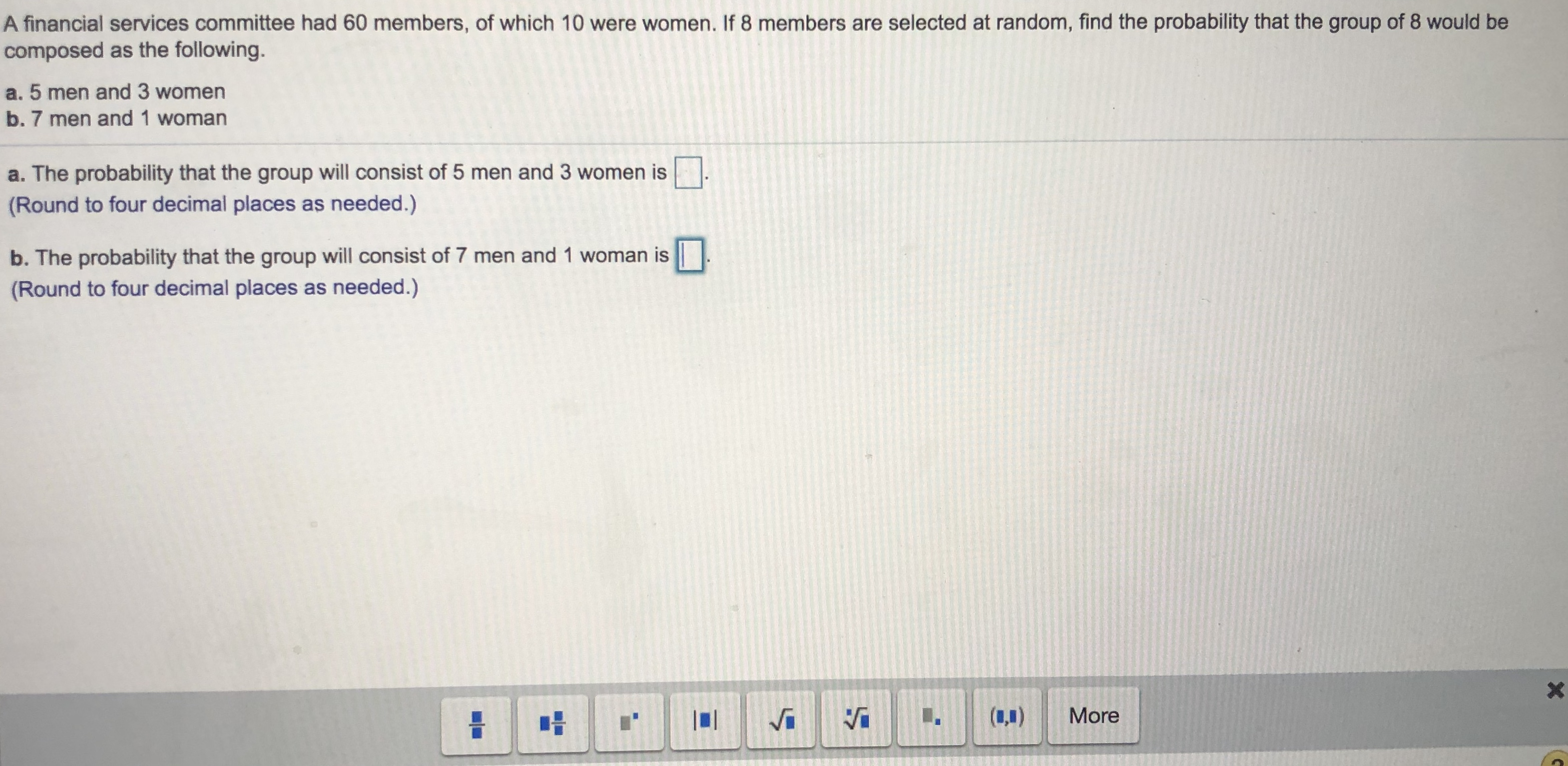# A financial services committee had 60 members, of which 10 were women. If 8 members are selected at random, find the probability that the group of 8 would becomposed as the following.a. 5 men and 3 womenb. 7 men and 1 womana. The probability that the group will consist of 5 men and 3 women is(Round to four decimal places as needed.)b. The probability that the group will consist of 7 men and 1 woman isRound to four decimal places as needed.)More

Question
1166 viewshelp_outlineImage TranscriptioncloseA financial services committee had 60 members, of which 10 were women. If 8 members are selected at random, find the probability that the group of 8 would be composed as the following. a. 5 men and 3 women b. 7 men and 1 woman a. The probability that the group will consist of 5 men and 3 women is (Round to four decimal places as needed.) b. The probability that the group will consist of 7 men and 1 woman is Round to four decimal places as needed.) More fullscreen
check_circle

Step 1

Concept of probability:

Probability deals with the likelihood of occurrence of a given event. The probability value lies between 0 and 1. An event with probability 1 is considered as certain event and an event with probability 0 is considered as an impossible event. The probability of 0.5 infers of having equal odds of occurring and not occurring of an event.

The general formula to obtain probability of an event A is,

P(A) = (number of favourable elements for event A)/(Total number of elements in the sample space).

The basic properties of probability are given below:

Step 2

a.

Find the probability that the group will consist of 5 men and 3 women:

The total number of persons in the financial services committee is 60.

The total number of women in the financial services committee is 10.

The total number of men in the financial services committee is 50.

The number of people selected at random in a group is 8.

Now, the requirement is that the selected group has to contain 5 men and 3 women.

That is, the investigator has to select 5 men from 50 men and 3 women from 10 women.

The sample space will be the number of ways in which 8 people are selected from 60 people.

The probability that the group will consist of 5 men and 3 women is obtained as 0.09937 from the calculation given below:

Step 3

b.

Find the probability that the group will consist of 7 men and 1 woman:

Here, the requirement is that the selected group has to contain 7 men and 1 woman.

That is, the investigator has to select 7 men from 50 men and 1 woman from 10 women.

The sample space will...

### Want to see the full answer?

See Solution

#### Want to see this answer and more?

Solutions are written by subject experts who are available 24/7. Questions are typically answered within 1 hour.*

See Solution
*Response times may vary by subject and question.
Tagged in
MathProbability

### Other